Students can Download Maths Chapter 4 Geometry Ex 4.3 Questions and Answers, Notes Pdf, Samacheer Kalvi 7th Maths Book Solutions Guide Pdf helps you to revise the complete Tamilnadu State Board New Syllabus and score more marks in your examinations.

## Tamilnadu Samacheer Kalvi 7th Maths Solutions Term 3 Chapter 4 Geometry Ex 4.3

Miscellaneous Practice problems

Question 1.
The bishop, in given picture of chess board, can move diagonally along dark squares. Describe the translations of the bishop after two moves as shown in the figure.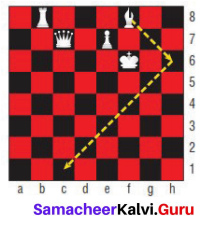Solution:
For first: 2 →, 2↓; For second move: 5 ←, 5↓

Question 2.
Write a possible translation for each of chess piece for a single move.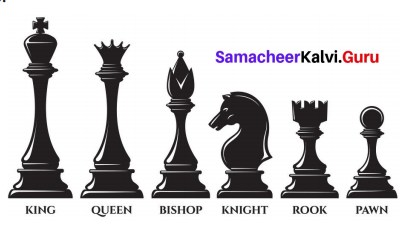Solution:
Pawn – 1 ↑ or 2↑
Rook – 1 to 8 ↑
Knight – 2 →,1 ↑or 2 ←,1 ↑ or 1 →,2 ↑or 1 ←,2↑
Bishop – 1 →,1 ↑ or 2 →,2↑or 3 →,3↑ or 4 →,4↑ or 5 → 5 ↑1 ←,1↑ or 2 ←,2↑or 3 ←,3↑or 4 ←,4↑ or 5 ← 5↑
Queen – 1 to 8 ,1 →, 1 ↑ or 2 →,2↑ or 3 → ,3↑or 4 → ,4↑or 5 →,5↑or 1 ←,↑1 or 2 ←,2 ↑or
3 ←,3 ↑ or 4 ←,4 ↑ or 5 ← 5↑
King – 1 → or ← or ↑Question 3.
Referring the graphic given, answer the following questions. Each bar of the category is made up of boy-girl-boy unit, (i) Which categories show a boy- girl-boy unit that is translation within the bar? (ii) Which categories show a boy-girl-boy unit that is reflected within the bar?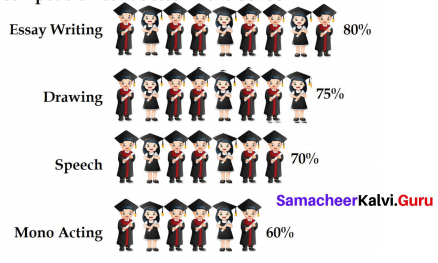Solution:
(i) Essay Writing category shows translation
(ii) Essay Writing and Mono Acting categories shows reflection

Question 4.
Given figure is a floor design in which the length of the small red equilateral triangle is 30 cm. All the triangles and hexagons are regular. Describe the translations in cm, represented by the (i) yellow line (ii) black line (iii) blue line.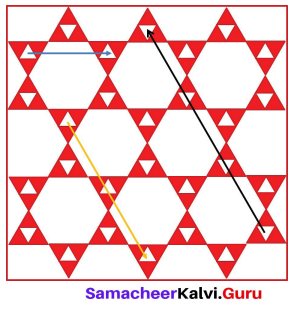Solution:
(i) 120cm →, 210cm ↓
(ii) 270cm ← ,330cm ↑
(iii) 150 cm →Question 5.
Describe the transformation involved in the following pair of figures (letters). Write translation, reflection or rotation.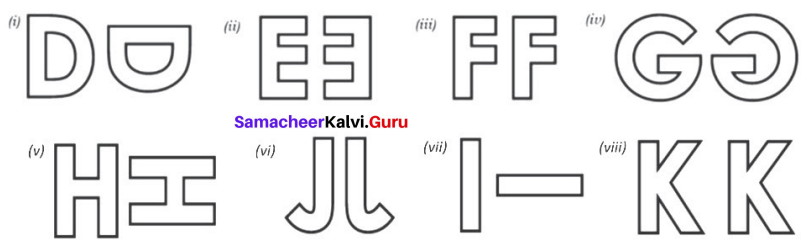Solution:
(i) rotation
(ii) reflection
(iii) translation
(iv) reflection
(v) rotation
(vi) reflection
(vii) rotation
(viii) translationChallenge problems

Question 1.
In chess, a knight can move only in an L-shaped pattern: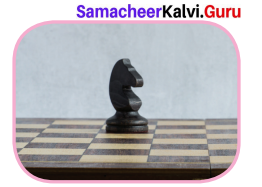• two vertical squares, then one horizontal square;
• two horizontal squares, then one vertical square;
• one vertical square, then two horizontal squares; or
• one horizontal square, then two vertical squares.

Write a series of translations to move the knight from g8 to g5 (at most two moves)
Solution:.
2 ←,1↓ and then 1 ←, 2↓ (or) 2 ←, 1↓and then 1 ← ,2↓

Question 2.
The pink shape is congruent to blue shape. Describe a sequence of transformations in which the blue shape is the image of pink shape.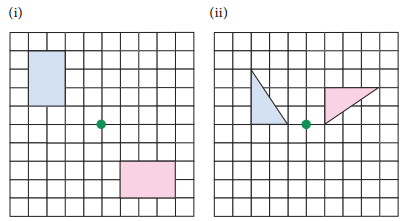Solution:
(i) Translation 3 ←, 5↑ and 90° counter clockwise rotation about the green point and translates 5 ←, 2↓,
(ii) Translation 2 ← 90° counter clockwise rotation about the green point and translates 2 ←, 2↓.Question 3.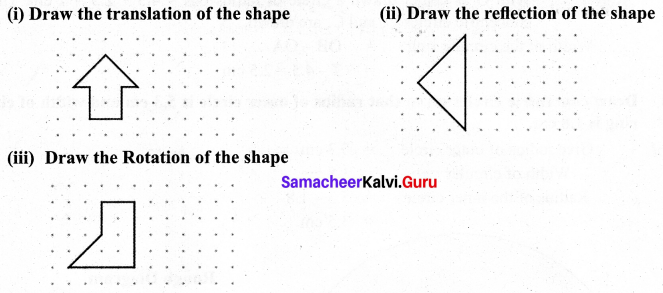Solution: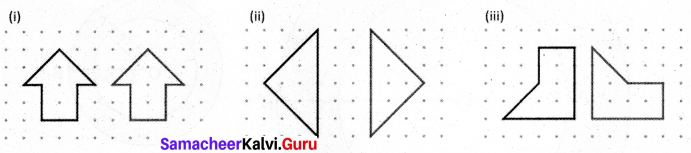Question 4.
Draw concentric circles given that radius of inner circle is 4.5 cm and width of circular ring is 2.5 cm.
Solution:
Give radius of inner circle = 4.5 cm
Width of circular ring is 2.5 cm
Radius of outer circle = 4.5 + 2.5 = 7 cm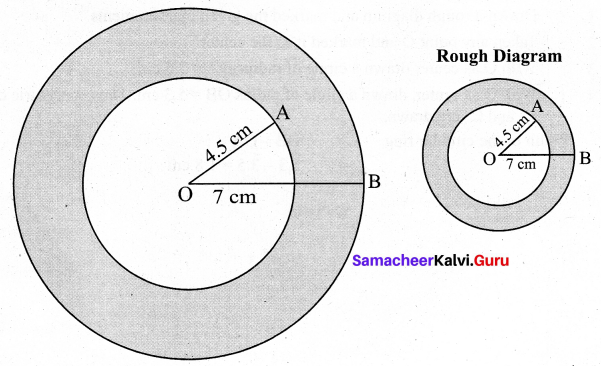Step 1 : Drawn a rough diagram and marked the given measurements
Step 2 : Taken any point O and marked it as the center.
Step 3 : With O as center and drawn a circle of radius OA = 4.5 cm.
Step 4 : With O as center drawn a circle of radius OB = 4.5 + 2.5 = 7 cm. Thus the concentric circles C1 and C2 are drawn.
Width of the circular ring = OB – OA
= 7 – 4.5 = 2.5 cmQuestion 5.
Draw concentric circles given that radius of outer circle is 5.3 cm and width of circular ring is 1.8 cm.
Solution:
Give radius of outer circle = 5.3 cm
Width of circular ring = 1.8 cm
Radius of the inner circle = 5.3 – 1.8
= 3.5 cm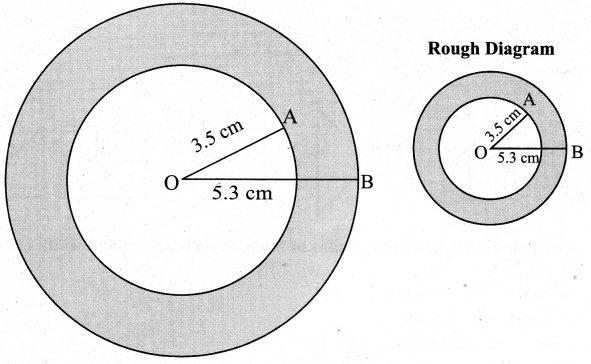Step 1: Drawn a rough diagram and marked the given measurements
Step 2 : Taken any point O and marked it as the center.
Step 3 : With O as center drawn a circle of radius OA =3.5 cm.
Step 4 : With O as center, drawn a circle of radius OB = 5.3 cm. Thus concentric circles
C1 and C2 are drawn.
Width of the circular ring = OB – OA
= 5.3 – 3.5 = 1.8 cm